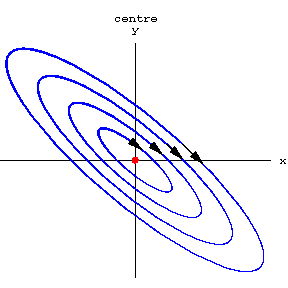# Centre

(More frequently spelled now as Center.)

2010 Mathematics Subject Classification: Primary: 34C15 Secondary: 34M35 [MSN][ZBL]

The topological type of a singular point of planar vector field, all of whose trajectories are closed (periodic).

## Contents

### Standard center

The equation of a mathematical pendulum, reduced to the system of two linear ordinary differential equations $$\dot x=y,\quad \dot y=-x, \qquad (x,y)\in(\R^2,0),$$ is the simplest example of a center, the so called linear center. Its trajectories form the concentric circles $x^2+y^2=r^2$ for all $r>0$.

A nonlinear center (or simply center) is any singularity of a planar vector field $v(x)$ which is topologically equivalent to the linear center.### Stability, integrability, reversibility

Centers are Lyapunov stable, but not asymptotically stable: all trajectories which start close enough to the singularity, never leave a specified (perhaps, larger) neighborhood of the point, yet do not tend to this point as $t\to\infty$ (the so called neutral stability).

If a vector field admits a local first integral which exhibits a strict local extremum, then the singular point is necessarily a center.

The inverse assertion, although true even in $C^\infty$-smooth category, has no practical meaning since a center-type singularity may have only flat $C^\infty$-smooth first integral (with the Taylor series vanishing identically). One important exception is the theorem due to H. Poincare and A. M. Lyapunov.

A real linear operator $\R^2\to\R^2$ is called elliptic, if its eigenvalues form a conjugate pair $\pm i\omega$, $\omega\ne 0$. A singular point of vector field $v(x)=Ax+\cdots$ is elliptic, if its linear part $A$ is an elliptic operator.

The Poincare--Lyapunov theorem asserts that if the vector field (polynomial or analytic) has an elliptic singularity, then it necessarily admits an analytic first integral with the nontrivial quadratic terms. If the linear part is the standard rotation as above, one can find the first integral starting with the terms $\tfrac12(x^2+y^2)+\cdots$. Recursive calculation of the higher coefficients of this integral gives an algorithm for eventual decision in the center-focus problem.

The linear center is a reversible vector field: the linear reflection $S:(x,y)\mapsto (x,-y)$ transforms the field into itself with the opposite sign, $\rd S\cdot v=-v\circ S$, changing thus the direction of the time variable. A singularity that is locally reversible by an invertible germ $S:(\R^2,0)\to(\R^2,0)$, are necessarily centers. The inverse is in general not true, but for singularities defined by a Pfaffian equation $y\rd y+\cdots=0$ each center is analytically reversible.

#### Complex analytic centers

In the complex domain there is no difference between the elliptic center (with the eigenvalues of the linear part $\pm i)$ and an integrable resonant saddle (with the eigenvalues $\pm 1$). Here integrability means that the vector field $v$, $$\dot x=x+\cdots,\qquad \dot y=-y+\cdots,\qquad (x,y)\in(\C^2,0)$$ has a holomorphic first integral $\mathscr O(\C^2,0)\owns f(x,y)=xy+\cdots$ (here and everywhere else the dots denote converging series with terms of order greater than all explicitly written). The saddle has a diagonal linear part which is more convenient for calculations, see center-focus problem.

How to Cite This Entry:
Centre. Encyclopedia of Mathematics. URL: http://encyclopediaofmath.org/index.php?title=Centre&oldid=30972
This article was adapted from an original article by A.F. Andreev (originator), which appeared in Encyclopedia of Mathematics - ISBN 1402006098. See original article